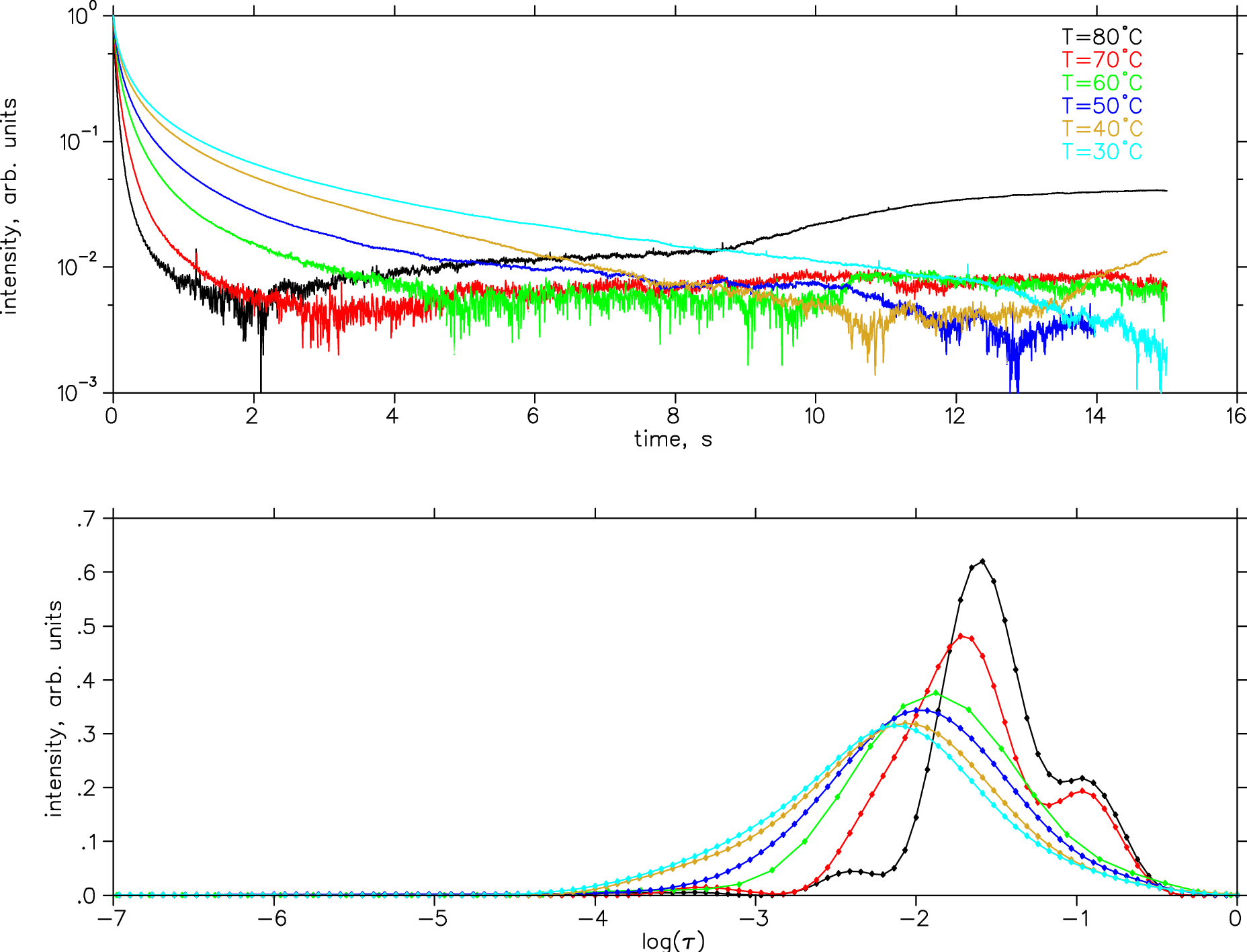# 6. Inverse theory methods¶

In :
%bash
ls /work/5P10/Inverse/

08_Sternin-reprint.pdf	60C.dat  expo_maketest.m	     pmma.eps
30C.dat			70C.dat  expo_maketest.sci	     pmma.png
40C.dat			80C.dat  expo.sci		     pmma.ps
50C.dat			expo.m	 inverse-theory-methods.pdf  SS97.pdf

In :
%plot --format svg -w 640 -h 480
## note: using svg format requires non-empty title to each graph,
## otherwise "no element found" error shows up. You can use title(" ");

In :
%%  expo_maketest.m
%    make f(t) + noise, for testing TR analysis of
%    a distribution of relaxation rates, g(r)
%
%  Completed: Dec.2005, Edward Sternin <edward dot sternin at brocku dot ca>
%  Contributions: Hartmut Schaefer
%  Revisions: 2018.02 ES converted to matlab/octave from SciLab
%
clear;

noise=0.05;     % noise as fraction of the max(f(t))
datafile=sprintf("noisy-%.2f.dat",noise);

t_steps=1000;   % t-domain signal has this many points,
t_min=1;        %  from this minimum,
t_max=1e5;      %  to this maximum
r_steps=500;    % r-grid has this many points,
r_min=1/t_max;  %  from this minimum,
r_max=1/t_min;  %  to this maximum

% Use an array of Gaussian peaks that make up the true g(r):
%peaks = [[0.5 0.20 0.04];[0.65 0.50 0.03];[0.75 0.80 0.03]];    % [amplitude center width]
peaks = [[0.5 0.20 0.04];[0.65 0.50 0.03]];   % [amplitude center width]
%peaks = [[0.6 0.35 0.1];[0.1 0.45 0.15]];    % [amplitude center width]

%  create a vector of r values, logarithmically spaced
r_scale = log(r_max)-log(r_min);
r = exp([log(r_min):r_scale/(r_steps-1):log(r_max)])'; % use a column vector

%  build up g(r) by adding Gaussian contributions from all peaks
g = zeros(length(r),1);
for k=1:length(peaks(:,1))
r0 = log(r_min) +  r_scale*peaks(k,2);
dr = r_scale*peaks(k,3);
g += peaks(k,1) * exp (- (log(r) - r0).^2 / (2 * dr^2));
end;

f1=figure(1); clf(1);
semilogx(r,g,'r-','LineWidth',2);
ylabel("g(r)");
xlabel("r");
title("Test distribution of relaxation rates");    % a bug: for svg graphics MUST have a title defined
FS = findall(f1,'-property','FontSize');
set(FS,'FontSize',14);
%print -dpng g.png

%  create a vector of t values, linearly spaced; use column vectors for f(t)
t = [t_min:(t_max-t_min)/(t_steps-1):t_max]';

%  generate time-domain data
f = (1/(length(t)-1)*g'*exp(-r*t'))';

%  normalize and add random noise
f /= max(f);
f += noise*(-0.5+rand(length(f),1));

f2=figure(2); clf(2);
plot(t,f,'g-');
%semilogy(t,f,'g-');
ylabel("f(t)");
xlabel("t");
title(sprintf("f(t) + %.1f%% random noise, saved in %s",100*noise,datafile));
FS = findall(f2,'-property','FontSize');
set(FS,'FontSize',14);
%print -dpng f.png

%system(sprintf("rm -rf %s",datafile));
f_of_t=[t f];
save("-ascii",datafile,"f_of_t");

g_of_r=[r g];
save("-ascii","true_g.dat","g_of_r");

In :
%% expo.m
%  multi-exponential inverse analysis of time decay curves
%    f(t) = \int g(r) exp(-r*t) dr
%
%  Notation:
%    r = vector or r values
%    n = number of points in r
%    g = vector of unknowns, g(r)
%    t = vector of t values
%    m = number of points in t
%    f = vector of measured data, f(t)
%    K = (m x n) kernel matrix
%    svd_cnt = keep only this many singular values
%    lambda = Tikhonov regularization parameter
%    datafile = (noisy) data, in two columns: (t,f)
%
%  Completed: Dec.2005, Edward Sternin <edward dot sternin at brocku dot ca>
%  Contributions: Hartmut Schaefer
%  Revisions: 2018.02 ES converted to matlab/octave from SciLab
%

clear;

function [g] = regularize(t,f,r,K,svd_n,lambda)
m=length(f);
n=length(r);
if (m < n)
error ("This code is not meant for underdetermined problems, need more data");
end;

%  SVD of the kernel matrix
[U,S,V]=svd(K);

nt=n;
%  if requested, truncate the number of singular values
if (svd_n > 0)
nt=min(n,svd_n);
nt=max(nt,2); % but not too few!
end;

%  Tikhonov regularization
sl=S(nt,nt);
for k=1:nt
sl(k,k)=S(k,k)/(S(k,k)^2+lambda);
end;

% return g(r)
g=V(1:n,1:nt)*sl*U(1:m,1:nt)'*f;

end;

%====================================================

datafile='noisy-0.05.dat';
r_steps=70;  % r-grid has this many points,
r_min=1e-6;  % from this minimum,
r_max=1;     % to this maximum
svd_cnt=0;   % set to 0 for no SVD truncation
lambda=5e-3; % set to 0 for no Tikhonov regularization

%  read in the time-domain data
t=f_of_t(:,1); % first column is time
f=f_of_t(:,2); % second column is f(t)

%  create a vector of r values, logarithmically spaced
r_inc = (log(r_max)-log(r_min)) / (r_steps-1);
r = exp([log(r_min):r_inc:log(r_max)]);

%  set up our kernel matrix, normalize by the step in r
K = exp(-t*r) * r_inc;

%  call the inversion routine
[g] = regularize(t,f,r,K,svd_cnt,lambda);

%  plot the original data f(t) and our misfit
f1=figure(1); clf(1);
hold on;
plot(t,f,'-');

misfit = f - K*g ;
Psi = sum(misfit.^2)/(length(f)-1);
scale=0.2*max(f)/max(misfit);
plot(t,scale*misfit,'-r');

title(sprintf("LS error norm = %f",Psi));
xlabel("t");
ylabel("f(t)");
legend("input data, f(t)",sprintf("misfit, x%.2f",scale));
FS = findall(f1,'-property','FontSize');
set(FS,'FontSize',14);
hold off;

%  separately, plot the result of the inversion
f2=figure(2); clf(2);
hold on;

r_true=g_of_r(:,1);
g_true=g_of_r(:,2);
r_inc_true=(log(max(r_true))-log(min(r_true)))/(length(r_true)-1);
semilogx(r_true,g_true/(sum(g_true)*r_inc_true),'r:','LineWidth',2);

semilogx(r,g/(sum(g)*r_inc),':o','MarkerSize',2);
title("Pseudo-inverse solution");
xlabel("r");
ylabel("g(r)");
legend("true g(r)",sprintf("%s, SVD=%d, \\lambda=%.2g",datafile,svd_cnt,lambda));
FS = findall(f2,'-property','FontSize');
set(FS,'FontSize',14);
ylim([-0.1 0.8])   % last-minute tweak, to avoid the legend
hold off;

%print -depsc result.eps


## Homework, due 2018-03-08¶

• Review the concept of SVD decomposition.
• Analyze the skeleton code of regularize(); make sure you understand every line.
• Modify the main code by adding appropriate loops, etc. to reproduce the results presented for the exponential example, including the L-curves, on the sample data provided in /work/5P10/test.dat
• Automate the optimum selection of parameter λ. One possible approach is to seek the value that corresponds to the shortest distance to the origin on the L-curve. Be efficient: vary the step size in λ depending on how strong the dependence on λ is.
• Optionally, use your program to analyze a real experimental data set (in /work/5P10/Inverse/).In :
%bash
ls /work/5P10/Inverse/*C.dat

/work/5P10/Inverse/30C.dat  /work/5P10/Inverse/60C.dat
/work/5P10/Inverse/40C.dat  /work/5P10/Inverse/70C.dat
/work/5P10/Inverse/50C.dat  /work/5P10/Inverse/80C.dat

In [ ]:

`